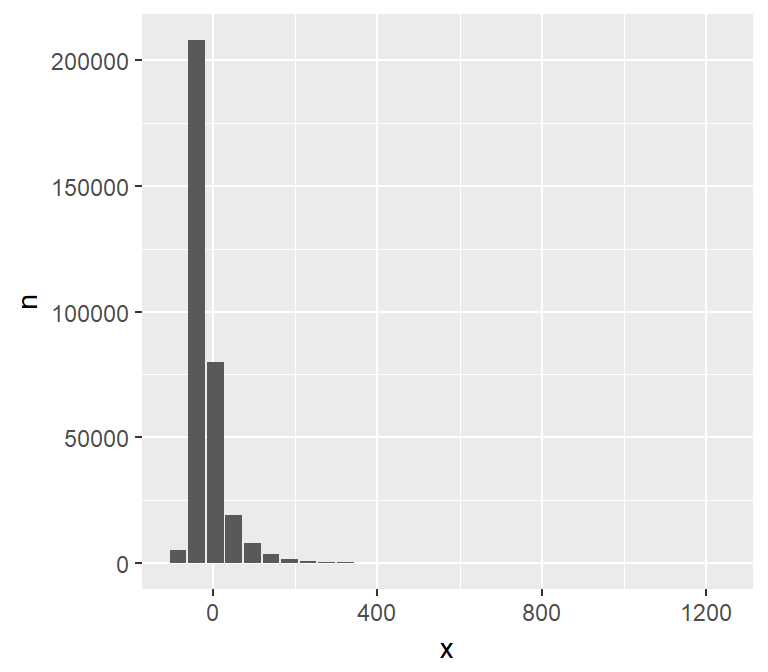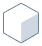# dbplot

Leverages `dplyr` to process the calculations of a plot inside a database. This package provides helper functions that abstract the work at three levels:

1. Functions that ouput a `ggplot2` object
2. Functions that outputs a `data.frame` object with the calculations
3. Creates the formula needed to calculate bins for a Histogram or a Raster plot

## Installation

``````# You can install the released version from CRAN
install.packages("dbplot")

# Or the the development version from GitHub:
install.packages("devtools")
devtools::install_github("edgararuiz/dbplot")``````

## Example

In addition to database connections, the functions work with `sparklyr`. A Spark DataFrame will be used for the examples in this README.

``````library(sparklyr)
sc <- spark_connect(master = "local", version = "2.1.0")
spark_flights <- copy_to(sc, nycflights13::flights, "flights")``````

## `ggplot`

### Histogram

By default `dbplot_histogram()` creates a 30 bin histogram

``````library(ggplot2)

spark_flights %>%
dbplot_histogram(sched_dep_time)``````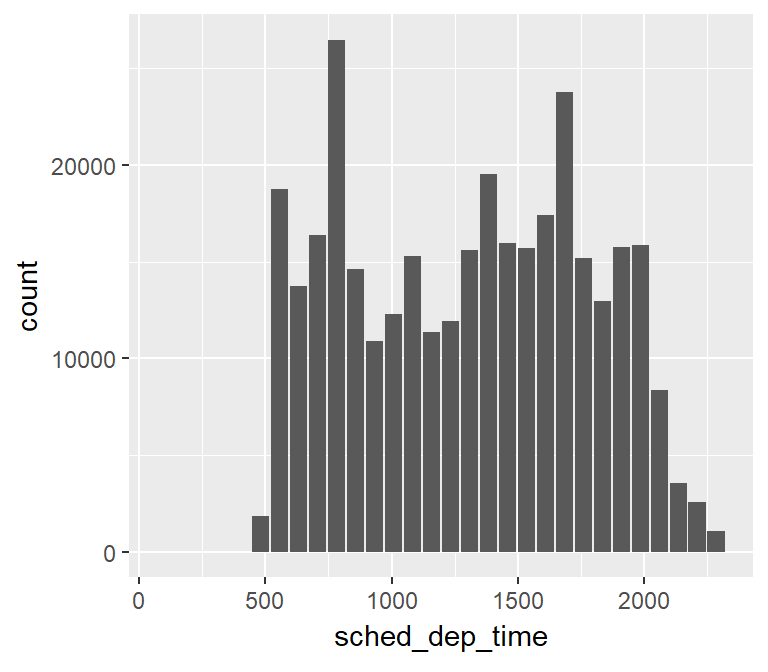Use `binwidth` to fix the bin size

``````spark_flights %>%
dbplot_histogram(sched_dep_time, binwidth = 200)``````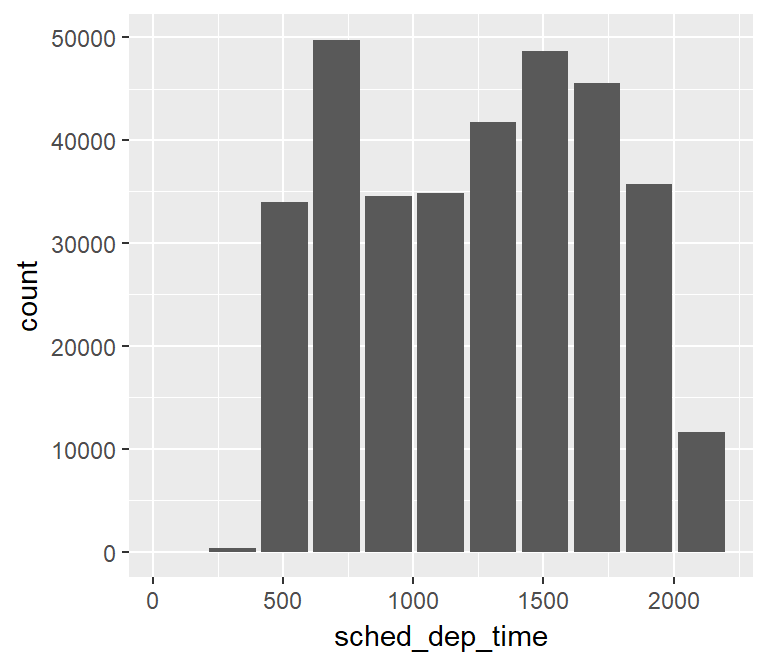Because it outputs a `ggplot2` object, more customization can be done

``````spark_flights %>%
dbplot_histogram(sched_dep_time, binwidth = 300) +
labs(title = "Flights - Scheduled Departure Time") +
theme_bw()``````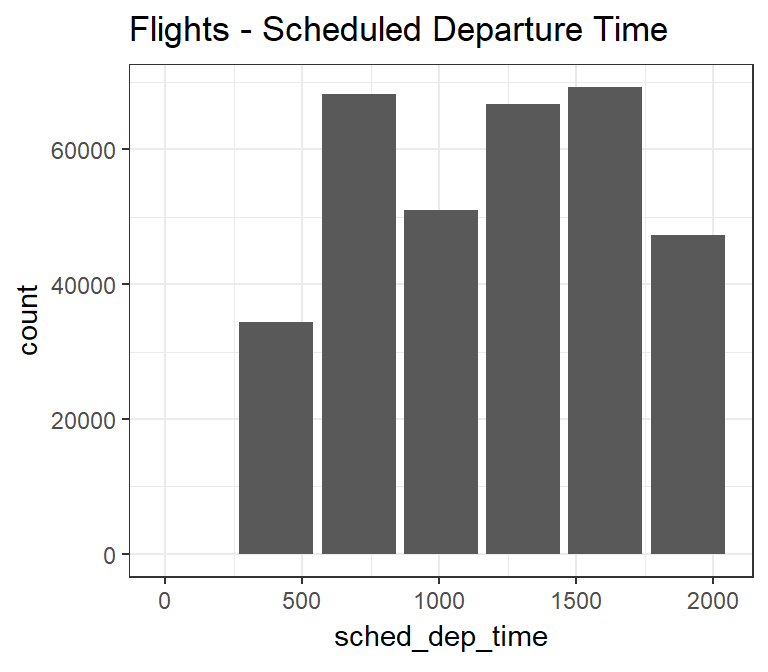### Raster

To visualize two continuous variables, we typically resort to a Scatter plot. However, this may not be practical when visualizing millions or billions of dots representing the intersections of the two variables. A Raster plot may be a better option, because it concentrates the intersections into squares that are easier to parse visually.

A Raster plot basically does the same as a Histogram. It takes two continuous variables and creates discrete 2-dimensional bins represented as squares in the plot. It then determines either the number of rows inside each square or processes some aggregation, like an average.

• If no `fill` argument is passed, the default calculation will be count, `n()`
``````spark_flights %>%
filter(!is.na(arr_delay)) %>%
dbplot_raster(arr_delay, dep_delay) ``````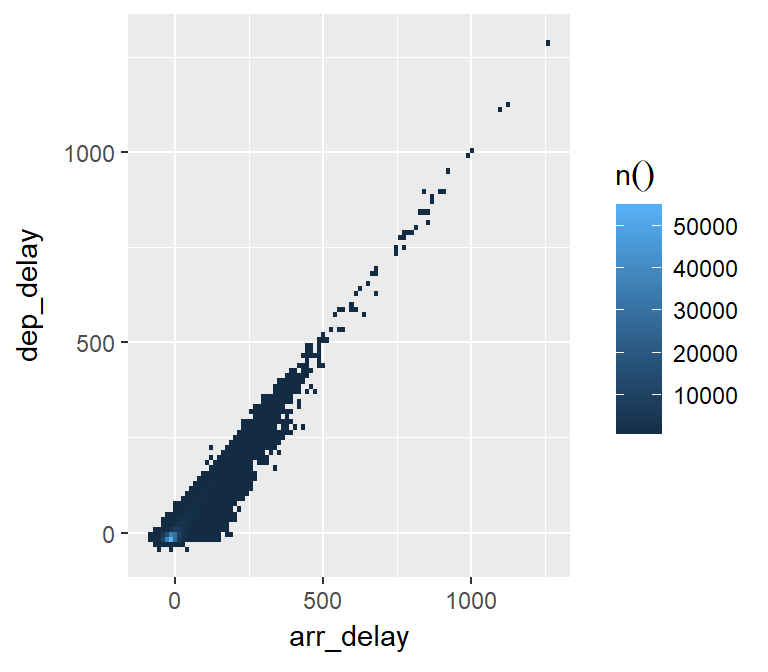• Pass an aggregation formula that can run inside the database
``````spark_flights %>%
filter(!is.na(arr_delay)) %>%
dbplot_raster(arr_delay, dep_delay, mean(distance, na.rm = TRUE)) ``````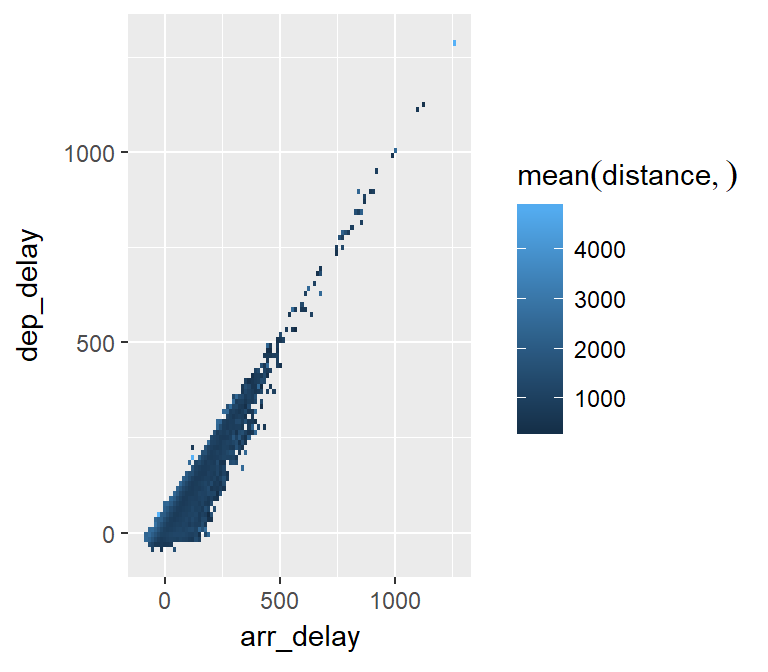• Increase or decrease for more, or less, definition. The `resolution` argument controls that, it defaults to 100
``````spark_flights %>%
filter(!is.na(arr_delay)) %>%
dbplot_raster(arr_delay, dep_delay, mean(distance, na.rm = TRUE), resolution = 500)``````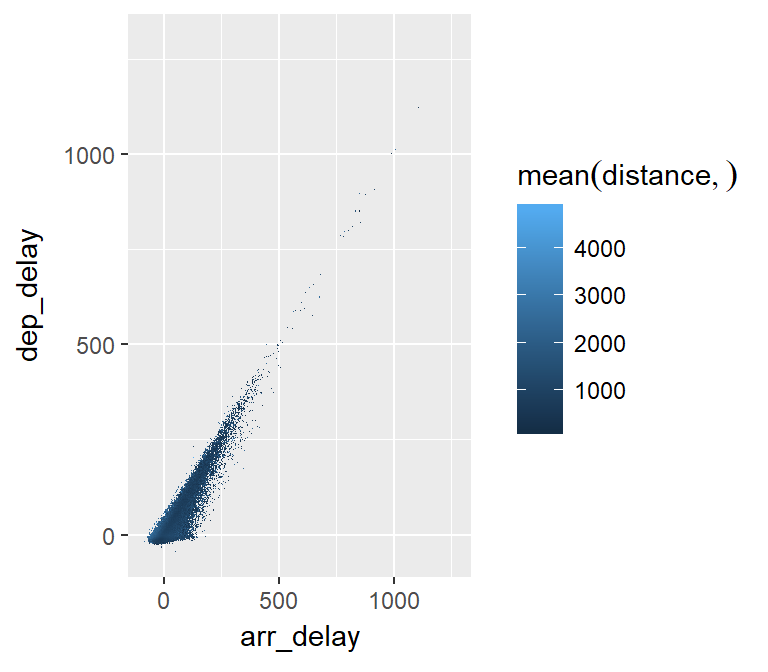### Bar Plot

• `dbplot_bar()` defaults to a tally() of each value in a discrete variable
``````spark_flights %>%
dbplot_bar(origin)``````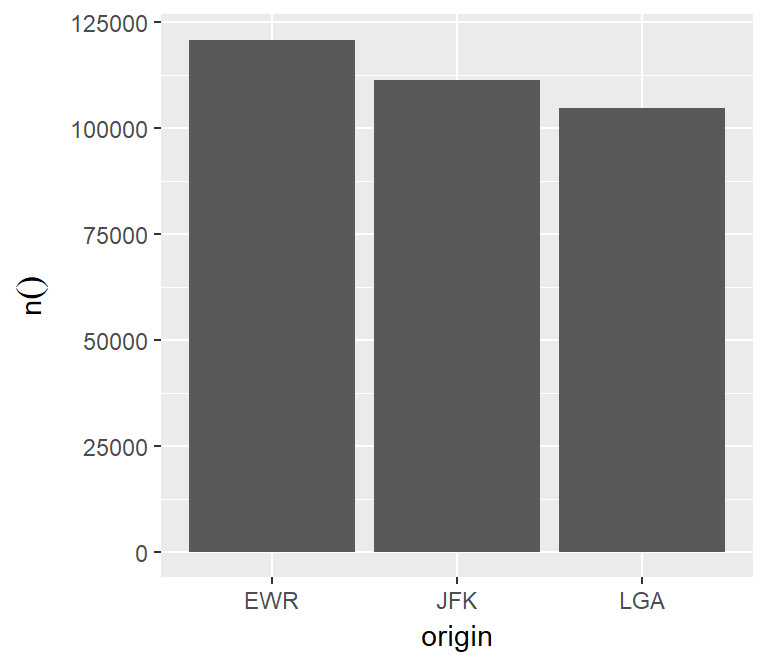• Pass a formula that will be operated for each value in the discrete variable
``````spark_flights %>%
dbplot_bar(origin, mean(dep_delay))``````
``````## Warning: Missing values are always removed in SQL.
## Use `AVG(x, na.rm = TRUE)` to silence this warning``````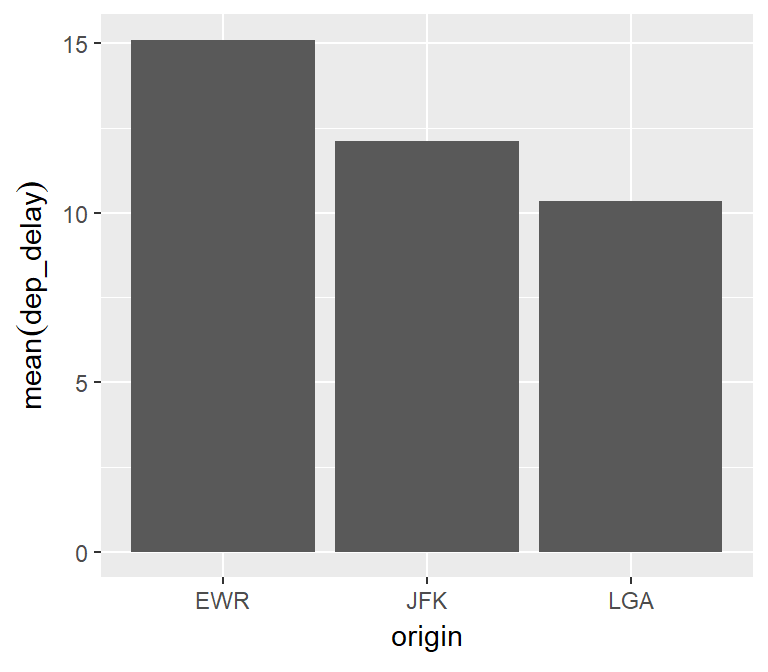### Line plot

• `dbplot_line()` defaults to a tally() of each value in a discrete variable
``````spark_flights %>%
dbplot_line(month)``````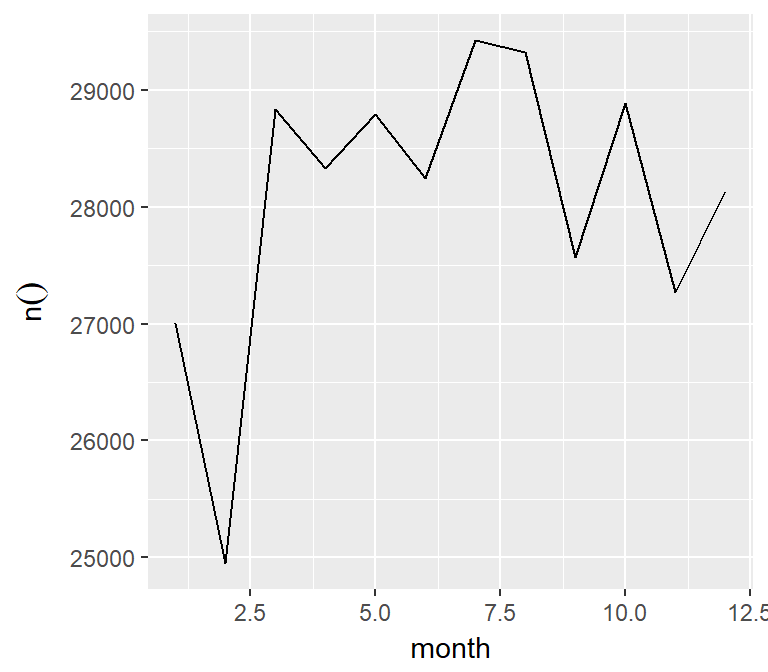• Pass a formula that will be operated for each value in the discrete variable
``````spark_flights %>%
dbplot_line(month, mean(dep_delay))``````
``````## Warning: Missing values are always removed in SQL.
## Use `AVG(x, na.rm = TRUE)` to silence this warning``````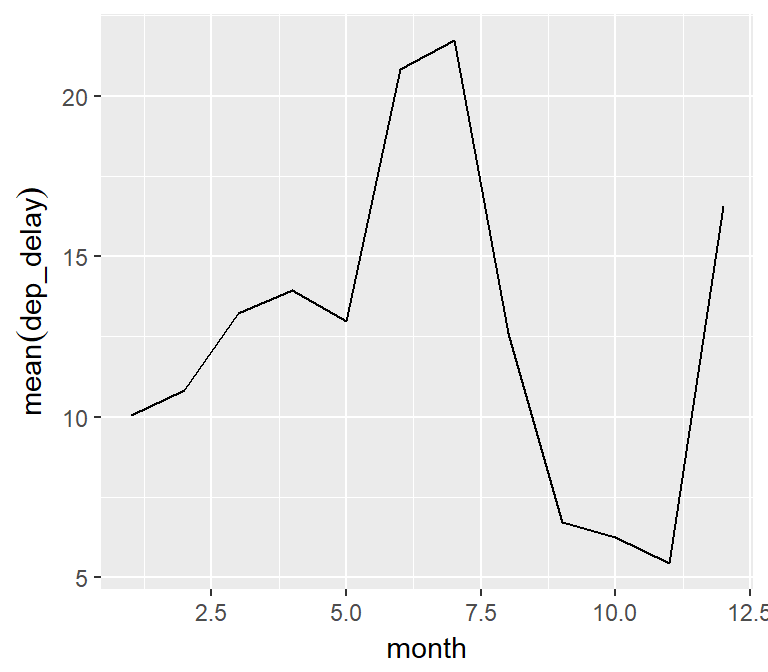### Boxplot

• It expects a discrete variable to group by, and a continuous variable to calculate the percentiles and IQR. It doesn’t calculate outliers. Currently, this feature works with sparklyr and Hive connections.
``````spark_flights %>%
dbplot_boxplot(origin, dep_delay)``````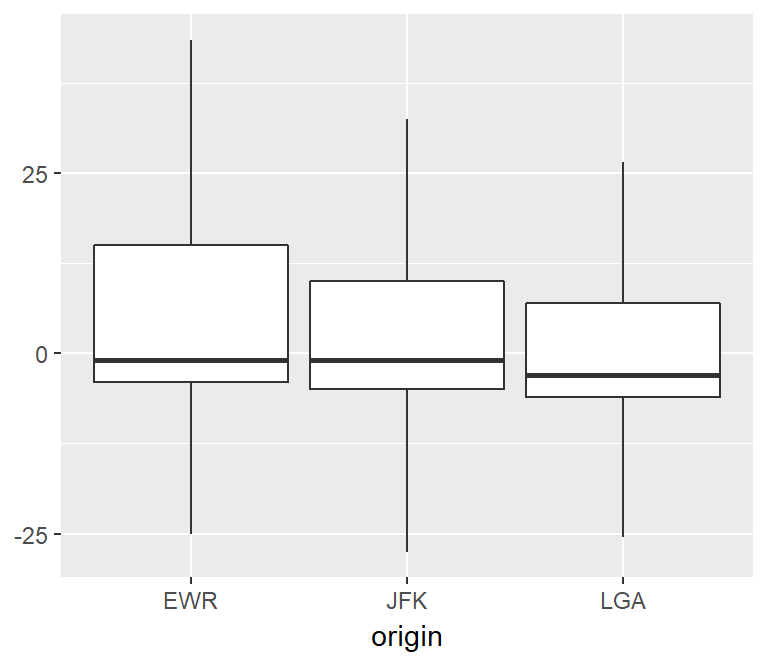## Calculation functions

If a more customized plot is needed, the data the underpins the plots can also be accessed:

1. `db_compute_bins()` - Returns a data frame with the bins and count per bin
2. `db_compute_count()` - Returns a data frame with the count per discrete value
3. `db_compute_raster()` - Returns a data frame with the results per x/y intersection
4. `db_compute_boxplot()` - Returns a data frame with boxplot calculations
``````spark_flights %>%
db_compute_bins(arr_delay) ``````
``````## # A tibble: 28 x 2
##    arr_delay     count
##        <dbl>     <dbl>
##  1      4.53  79784
##  2   - 40.7  207999
##  3     95.1    7890
##  4     49.8   19063
##  5    819         8.00
##  6    140      3746
##  7    321       232
##  8    231       921
##  9   - 86.0    5325
## 10    186      1742
## # ... with 18 more rows``````

The data can be piped to a plot

``````spark_flights %>%
filter(arr_delay < 100 , arr_delay > -50) %>%
db_compute_bins(arr_delay) %>%
ggplot() +
geom_col(aes(arr_delay, count, fill = count))``````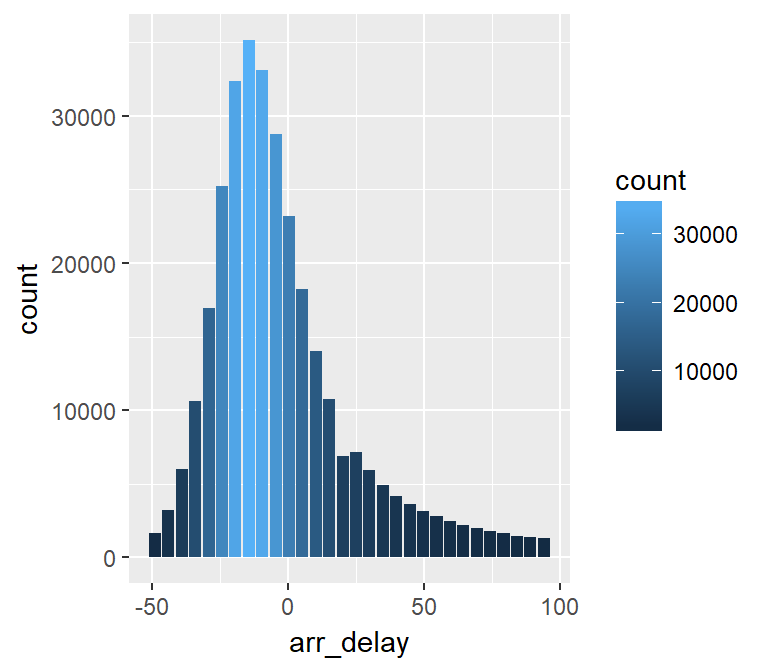## `db_bin()`

Uses ‘rlang’ to build the formula needed to create the bins of a numeric variable in an un-evaluated fashion. This way, the formula can be then passed inside a dplyr verb.

``db_bin(var)``
``````## (((max(var, na.rm = TRUE) - min(var, na.rm = TRUE))/(30)) * ifelse((as.integer(floor(((var) -
##     min(var, na.rm = TRUE))/((max(var, na.rm = TRUE) - min(var,
##     na.rm = TRUE))/(30))))) == (30), (as.integer(floor(((var) -
##     min(var, na.rm = TRUE))/((max(var, na.rm = TRUE) - min(var,
##     na.rm = TRUE))/(30))))) - 1, (as.integer(floor(((var) - min(var,
##     na.rm = TRUE))/((max(var, na.rm = TRUE) - min(var, na.rm = TRUE))/(30))))))) +
##     min(var, na.rm = TRUE)``````
``````spark_flights %>%
group_by(x = !! db_bin(arr_delay)) %>%
tally()``````
``````## # Source:   lazy query [?? x 2]
## # Database: spark_connection
##          x         n
##      <dbl>     <dbl>
##  1    4.53  79784
##  2 - 40.7  207999
##  3   95.1    7890
##  4   49.8   19063
##  5  819         8.00
##  6  140      3746
##  7  321       232
##  8  231       921
##  9 - 86.0    5325
## 10  186      1742
## # ... with more rows``````
``````spark_flights %>%
filter(!is.na(arr_delay)) %>%
group_by(x = !! db_bin(arr_delay)) %>%
tally()%>%
collect %>%
ggplot() +
geom_col(aes(x, n))``````